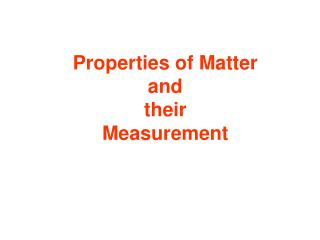Download PresentationProperties of Matter and their MeasurementProperties of Matter and their Measurement - PowerPoint PPT Presentation

Download PresentationProperties of Matter and their Measurement
An Image/Link below is provided (as is) to download presentation

Download Policy: Content on the Website is provided to you AS IS for your information and personal use and may not be sold / licensed / shared on other websites without getting consent from its author. While downloading, if for some reason you are not able to download a presentation, the publisher may have deleted the file from their server.

- - - - - - - - - - - - - - - - - - - - - - - - - - - E N D - - - - - - - - - - - - - - - - - - - - - - - - - - -
Presentation Transcript

1. Properties of MatterandtheirMeasurement

2. All measurements in science are done using the International System of Units (SI). This is basically the metric system. This system is easy to use because it is based on multiples of 10. Each SI measurement has a base unit. Prefixes are used with the base unit to make different size units. A measurement must have a number and a unit or it has no meaning.

3. Length – The distance between two points. The base unit of length is the meter. 1 cm 10 mm

4. 0.6 cm 6 mm 1.2 cm 12 mm 3.3 cm 33 mm 2.8 cm 28 mm

5. Volume – The amount of space an object takes up. • For solids, think of size. How big? • For liquids, think of amount. How much? The base unit for liquid volume is the liter. Liquids and solids are measured differently so we will look at them separately.

6. Liquid Volume There are many devices to measure liquid volume such as beakers, flasks and graduated cylinders. To make an exact measurement a graduated cylinder should be used.

7. When measuring the volume of a liquid: • Place the container on a flat, level surface. • Read it at eye level. • If there is a dip at the top of the liquid, read the bottom of the dip. This dip is called the meniscus

8. When measuring the volume of a solid: If the object has a regular geometric shape, a math formula is used. For example, if it is a box, you would use (length)(width)(height). 10cm V = (l)(w)(h) = (10cm)(2cm)(5cm) = 100cm3 5cm 2 cm The unit for solid volume is always cubed.

9. If the solid has no regular geometric shape, you can use water displacement to find its volume. You calculate the amount of water displaced by the solid. Example: 1. You put 50 ml of water in a graduate. 2. You put an object in and the water goes up to 72 ml. 3. Subtract to get the volume of the solid. (72 – 50 = 22ml)

10. If you are measuring the volume of a solid, it does not sound right to say the volume is 22 ml. Solid volume is always a cubed number, like 22 cm3. However, 1ml is equal in volume to 1 cm3. Therefore, you can automatically say 22 cm3 instead of 22 ml.

11. Mass – the amount of matter in an object. Mass is measured on a balance, like a triple beam balance. Weight–a measure of gravitational pull on an object. Weight is measured with a spring scale.

12. The base unit for mass is grams. A penny is 1 gram of mass. A nickel is 5 grams of mass. Weight is measured in a unit called newtons which we will learn about later. Weight changes if gravity changes but mass does not. The measurement on a spring scale will change with gravity but the measurement on a balance will not.

13. Density – the amount of mass in a certain amount of volume. The mass and volume of a substance will change depending on how much is present, but the density of the substance will always be the same. Density is given as the number of grams in each cubic centimeter. (g/cm3)

14. To find the density of a substance, youmust know its mass and volume. Example: An object has a mass 50g and a volume of 10cm3. Density (D) = mass(m)/ volume(v) = 50g/10cm3 = 5 g/cm3

15. The unit for density is a combination of the units, grams and cubic centimeters. Any unit made of a combination of units is called a derived unit. Any unit multiplied by itself is a derived unit. (cm)(cm)cm) = cm3 Therefore, cm3 is a derived unit. The density of water is 1g/cm3.

16. The temperature of a substance is measured with a thermometer. There are three temperature scales:

17. The SI unit of temperature is the Kelvin.(K) “0” on the Kelvin scale is called absolute 0. It is the temperature at which all motion of the molecules stops so it is the coldest possible temperature. A change of one degree Celsius is equal to a change of one Kelvin. There are no degrees with the Kelvin scale. The Si unit of time is the second. (s)